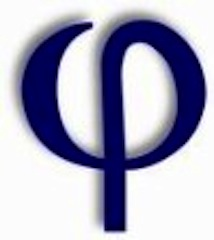# The Strong Goldbach Conjecture: An Equivalent StatementSome problems in mathematics remain buried deep in the catacombs of slow progress.   Whether in Algebra, Topology, Discrete Math, or Analysis, mind-stretching mysteries await to be discovered beyond the boundaries of former thought.  Frequently, solutions to such problems come from a clever combination of previously known results.   In conjunction with this concept, it is often helpful to reformulate open problems into equivalent statements to gain different perspectives.

As an example, the unsolved Strong Goldbach Conjecture, that proposes every even integer greater than 2 is the sum of two prime numbers, can be reformulated into an equation involving Euler’s Totient function.   By definition, two integers$m$ and$n$ are said to be relatively prime if the greatest common divisor of$m$ and$n$ is 1, denoted$gcd(m,n) = 1$.  For instance, let$m$ = 3 and$n$ = 5.  Since there are no divisors greater than 1 that is shared between 3 and 5, notably both being prime numbers, the$gcd(3,5) = 1$.  Therefore, 3 and 5 are said to be relatively prime.   With this in mind, Euler’s Totient function, denoted$\phi (n)$, is the total number of positive integers less than or equal to$n$ such that they are relatively prime to$n$.

Intuitively, for every prime number p and integer$k < p$,$gcd(p,k) = 1$ , and therefore$\phi (p)$  =$p$  – 1.    Given this, the statement of the Strong Goldbach Conjecture can be restated as: for every integer$n \geq 1$, there exists primes p and q such that$\phi(p) + \phi(q) = 2n.$ The mathematicians Paul Erdős and Leo Moser further asked whether or not there exists any integers p and q, not necessarily prime, such that the reformulated statement holds.

In considering the proposition of Erdős  and Moser, such questions provide a good starting point for solving the harder problem of considering p and q such that they are necessarily prime.   It does so by seeking to expose the nature of the equation in general.  Reformulations of this type provide a digging tool that could one day unearth a proof to the Strong Goldbach Conjecture, and other unsolved conundrums, from the catacombs of mathematical lethargy.Avery Carr is a senior analyst and past senior editor for the American Mathematical Society Grad Blog. He and his wife, Alison, live in Olive Branch, MS.
This entry was posted in AMS, Math, Mathematics in Society, Uncategorized. Bookmark the permalink.

### 1 Response to The Strong Goldbach Conjecture: An Equivalent Statement

1.Jan says:

In May I published “The binary Goldbach conjecture paper” on ScienceOpen preprints. If you would like to peruse it, the DOI is:

DOI: 10.14293/S2199-1006.1.SOR-.PPIU46T.v1

Regards J. Feliksiak